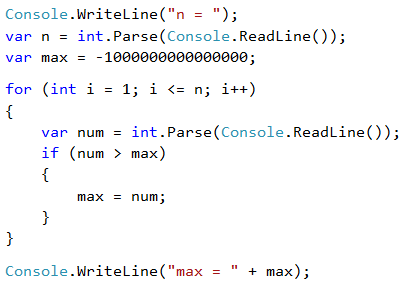# Problem: Max Number

Write a program that enters n integers (n > 0) and finds the max number among them (the largest number). The first line of the input, specifies the number of integers n. The next n lines hold the integers, one per line. Examples:

Input Output
2
100
99
100
3
-10
20
-30
20
4
45
-20
7
99
99
1
999
999
2
-1
-2
-1

## Video: Largest Number

Watch this video lesson to learn how to find the largest number among a sequence of number: https://youtu.be/KErfOdOuezE.

## Hints and Guidelines

We will first enter one number n (the number of integers that are about to be entered). We assign the current maximum max an initial neutral value, for example -10000000000000 (or int.MinValue). Using a for loop that is iterated n-1 times, we read one integer num on each iteration. If the read number num is higher than the current maximum max, we assign the value of the num to the max variable. Finally, in max we must have stored the highest number. We print the number on the console.